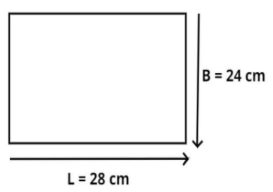Courses
Courses for Kids
Free study material
Free LIVE classes
More# A picture frame has dimensions $24cm \times 28cm$. Find the area of the picture.Verified
328.5k+ views
Hint: In this question first draw the diagram it will give us a clear picture of what we have to find out, and the length and breadth of the picture frame is different so it is in a rectangular shape, so use this concept to reach the solution of the question.

Given dimensions are $24cm \times 28cm$.Now from figure it is clear that the picture frame is in a rectangular shape,
So, the length of the picture frame is 28 cm and the breadth of the picture frame is 24 cm.
Now as we know that the area of rectangular shape = $L \times B$ square unit
So, area (A) of the picture frame is
$A = 28cm \times 24cm \\ \Rightarrow A = 28 \times 24{\text{ c}}{{\text{m}}^2} \\$
Now multiply 24 and 28 together we have,
$\Rightarrow A = 672{\text{ c}}{{\text{m}}^2}$
So, this is the required area of the picture frame.

Note: In such types of questions the key concept we have to remember is that the area of the rectangular picture frame is the direct multiplication of length and breadth, so multiply the given dimensions as above we will get the required area of the picture frame which is the required answer.
Last updated date: 05th Jun 2023
Total views: 328.5k
Views today: 2.85k